1-414-299-3896 Outside the U.S.

Our goal is to provide a variety of choices for any flow measurement application at industry leading prices.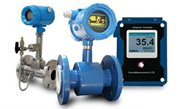# Thermal Measuring Principle

## Thermal Technology

Thermal mass flow meters are gas flow meters based on the relationship between convection heat transfer and mass flow. There are two types of thermal flow meters: rate of heat loss flowmeters and temperature rise flowmeters.

## Rate of Loss Flowmeter

Rate of heat loss thermal flow meters measure the rate of heat loss to the flow stream from a heated element such as a resistance wire, thermistor, thermocouple, or thin film sensor.

Governing Equation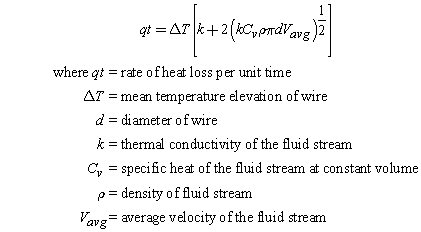In this equation,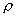Vavg, qt, and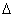T are the unknowns, because they change with time while the other variables are known. However, qt andT can be obtained through measuring devices, leaving in the product ofand Vavg and cross section area of the pipe.

## Temperature Rise Thermal Flow Meter

Temperature Rise thermal flow meters measure the temperature changes of the flow as it passes through a heat source.

Governing Equation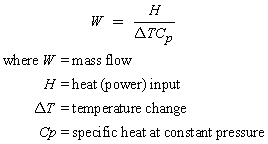W andT are unknowns in this equation.T can be measured. W can therefore be calculated.

Note that thermal flow meters are gas flow meters only and can be used as liquid flow switch but cannot be used as liquid flow meter due to the sudden drop inT because of the higher cooling rate of liquids compared to gases.  The higher cooling rate of liquids prevents the transmitter from calculating an adequate flow range in liquids.